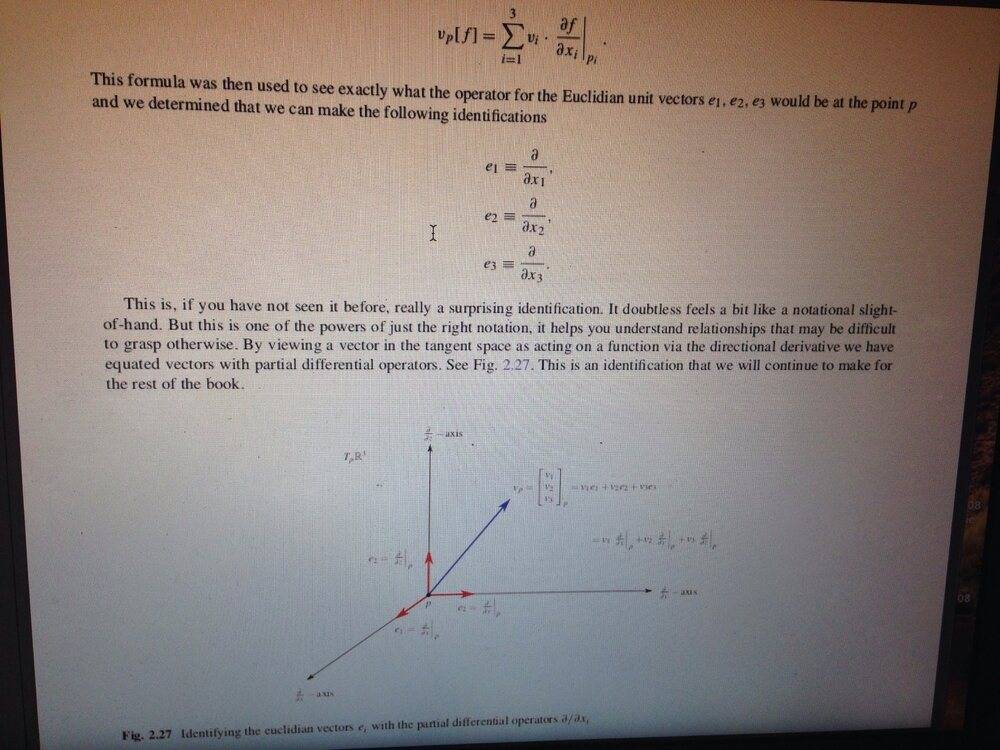# Directional Derivatives of a vector ----gradient of f(P)----

• I
• Ishika_96_sparkles

#### Ishika_96_sparkles

TL;DR Summary
Definition: Let f be a differentiable real-valued function on ##\mathbf{R}^3##, and let ##\mathbf{v}_P## be a tangent vector to it. Then the following number is the derivative of a function w.r.t. the tangent vector

$$\mathbf{v}_p[\mathit{f}]=\frac{d}{dt} \big( \mathit{f}(\mathbf{P}+ t \mathbf{v}) \big)|_{t=0}$$

Then there is this

Lemma: If ##\mathbf{v}_p= (v_1,v_2,v_3)_P## is a tangent vector to ##\mathbf{R}^3##, then

$$\mathbf{v}_p[\mathit{f}]= \sum_i v_i \frac{d \mathit{f}}{dx_i}(P)$$

1) How does this gradient term come into the picture? Since, $\mathit{f}(\mathbf{P}+ t \mathbf{v})$, do we write the argument as $\mathbf{x}(t)$ and then apply the chain-rule in the definition?

2) Could this be thought of as a dot-product ##\mathbf{v}_P \,. \, \nabla \mathit{f}|_p ##?

Last edited:
•troglodyte

2) is solved. Indeed its a dot product between the tangent vector and the basis frames.

The question 1) is solved too. The intuition was correct that it has to do with $x_i (t)= p_i+ t v_i$. The curve along this vector is parameterized by t and the coordinates of the space are a function of this parameter i.e. $\{x_1(t),x_2(t),x_3(t)\}$. Finally, the chain rule is applied on the real-valued function f as

$$\sum_{i=1}^3 \frac{df}{dx_i}\frac{dx_i}{dt}=\sum_{i=1}^3\frac{df}{dx_i}\, v_i$$

I was reading from some pdf notes, earlier. Now, I've found the book to learn it properly.
It is the Differential Geometry by Erwin Kreyszig.

Last edited:
•troglodyte
Maybe you can also try Hubbard ,Vector Calculus,lineare Algebra and differential forms.It is didactically one of the best books on this level.

Here is nice picture to the Lemma:Last edited:
•Ishika_96_sparkles
Maybe you can also try Hubbard ,Vector Calculus,lineare Algebra and differential forms.It is didactically one of the best books on this level.

Here is nice picture to the Lemma:

View attachment 264020

Simply WOW!

I just saw the preview and fell in love wit the pedagogical style. Thank you so much for such a wonderful suggestion.•troglodyte
Simply WOW!

I just saw the preview and fell in love wit the pedagogical style. Thank you so much for such a wonderful suggestion.I am glad that this Suggestion could help you•Ishika_96_sparkles